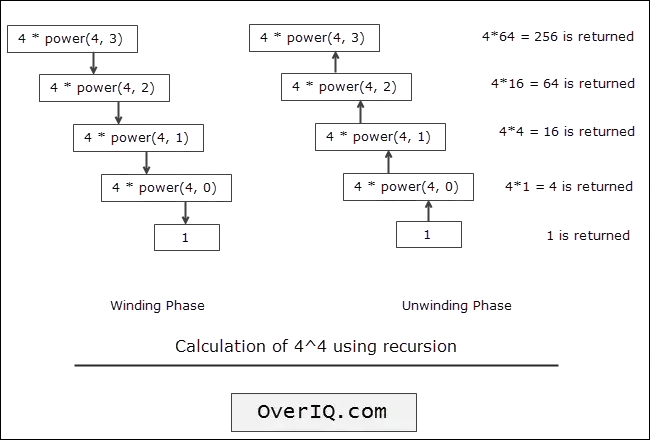# C Program to calculate the power using recursion

Last updated on September 24, 2020

The following is a C program to calculate the power using recursion:

  1 2 3 4 5 6 7 8 9 10 11 12 13 14 15 16 17 18 19 20 21 22 23 24 25 26 27 28 29 30 31 32 33 34 35 36 37 38 39 40 41 42 /*************************************************** Program to calculate the power using recursion * * Enter base: 2 * Enter exponent: 5 * 2^5 = 32 ***************************************************/ #include // include stdio.h library int power(int, int); int main(void) { int base, exponent; printf("Enter base: "); scanf("%d", &base); printf("Enter exponent: "); scanf("%d", &exponent); printf("%d^%d = %d", base, exponent, power(base, exponent)); return 0; // return 0 to operating system } int power(int base, int exponent) { //base condition if(exponent == 0) { return 1; } else { // recursive call return base * power(base, exponent - 1); } } 

Expected Output: 1st run:

 1 2 3 Enter base: 2 Enter exponent: 0 2^0 = 1 

2nd run:

 1 2 3 Enter base: 4 Enter exponent: 4 4^4 = 256 

## How it works #

The following figure shows how the recursive evaluation of $$4^4$$ takes place.